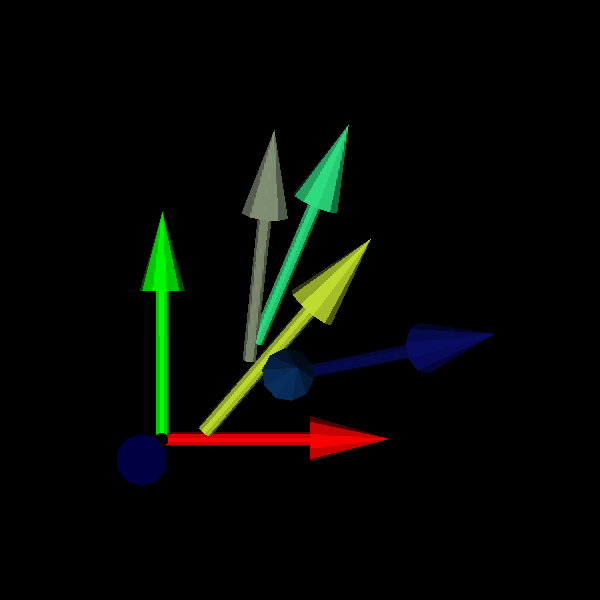# Fury Arrow Actor#

This example shows how to use the arrow actor.

```import numpy as np
from fury import window, actor
```

First thing, you have to specify centers, directions, and colors of the arrow(s)

```centers = np.zeros([3, 3])
```

np.identity is the same as specifying x, y, and z directions.

```dirs = np.identity(3)
colors = np.identity(3)
scales = np.array([2.1, 2.6, 2.5])
```

The below arrow actor is generated by repeating the arrow primitive.

```arrow_actor = actor.arrow(centers, dirs, colors=colors, scales=1.5)
```

repeating what we did but this time with random centers, directions, and colors.

```cen2 = np.random.rand(5, 3)
dir2 = np.random.rand(5, 3)
cols2 = np.random.rand(5, 3)

arrow_actor2 = actor.arrow(cen2, dir2, colors=cols2, scales=1.5)

scene = window.Scene()
```

Adding our Arrow actors to scene.

```scene.add(arrow_actor)